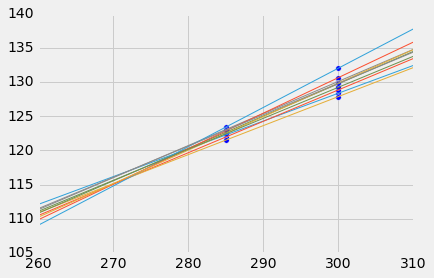# 十四、回归的推断

## 回归模型

• `x``y`之间的关系是完全线性的。我们看不到这个“真实直线”，但它是存在的。
• 散点图通过将线上的点垂直移动，或上或下来创建，如下所示：
• 对于每个`x`，找到真实直线上的相应点（即信号），然后生成噪声或误差。
• 误差从误差总体中带放回随机抽取，总体是均值为 0 的正态分布。
• 创建一个点，横坐标为`x`，纵坐标为“`x`处的真实高度加上误差”。
• 最后，从散点图中删除真正的线，只显示创建的点。

``````# The true line,
# the points created,
# and our estimate of the true line.
# Arguments: true slope, true intercept, number of points

draw_and_compare(4, -5, 10)
``````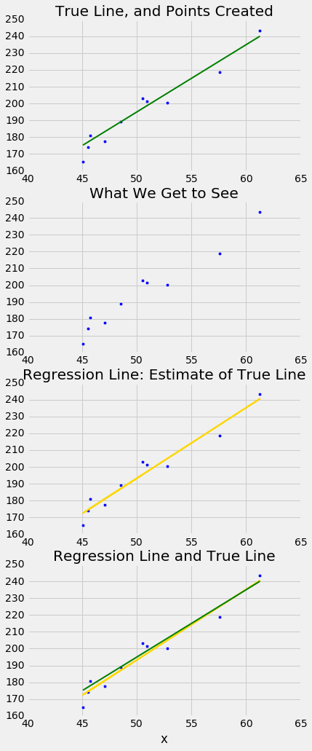## 真实斜率的推断

``````correlation(baby, 'Gestational Days', 'Birth Weight')
0.40754279338885108
``````

``````slope(baby, 'Gestational Days', 'Birth Weight')
0.46655687694921522
``````

### 自举散点图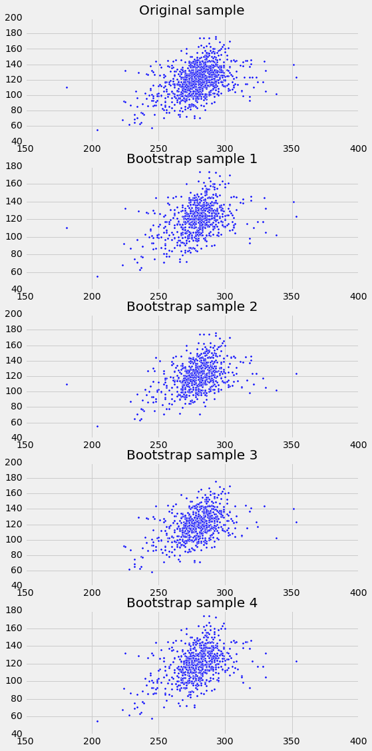### 估计真实斜率

``````slopes = make_array()
for i in np.arange(5000):
bootstrap_sample = baby.sample()
bootstrap_slope = slope(bootstrap_sample, 'Gestational Days', 'Birth Weight')
slopes = np.append(slopes, bootstrap_slope)
Table().with_column('Bootstrap Slopes', slopes).hist(bins=20)
``````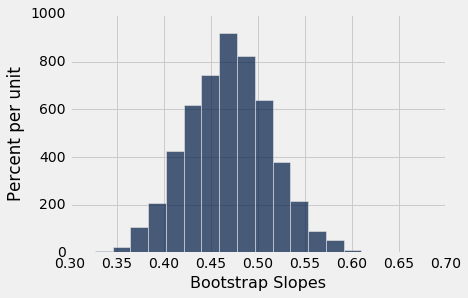``````left = percentile(2.5, slopes)
right = percentile(97.5, slopes)
left, right
(0.38209399211893086, 0.56014757838023777)
``````

### 用于自举斜率的函数

``````def bootstrap_slope(table, x, y, repetitions):

# For each repetition:
# Bootstrap the scatter, get the slope of the regression line,
# augment the list of generated slopes
slopes = make_array()
for i in np.arange(repetitions):
bootstrap_sample = table.sample()
bootstrap_slope = slope(bootstrap_sample, x, y)
slopes = np.append(slopes, bootstrap_slope)

# Find the endpoints of the 95% confidence interval for the true slope
left = percentile(2.5, slopes)
right = percentile(97.5, slopes)

# Slope of the regression line from the original sample
observed_slope = slope(table, x, y)

# Display results
Table().with_column('Bootstrap Slopes', slopes).hist(bins=20)
plots.plot(make_array(left, right), make_array(0, 0), color='yellow', lw=8);
print('Slope of regression line:', observed_slope)
print('Approximate 95%-confidence interval for the true slope:')
print(left, right)
``````

``````bootstrap_slope(baby, 'Gestational Days', 'Birth Weight', 5000)
Slope of regression line: 0.466556876949
Approximate 95%-confidence interval for the true slope:
0.378663152966 0.555005146304
````````````scatter_fit(baby, 'Maternal Height', 'Birth Weight')
``````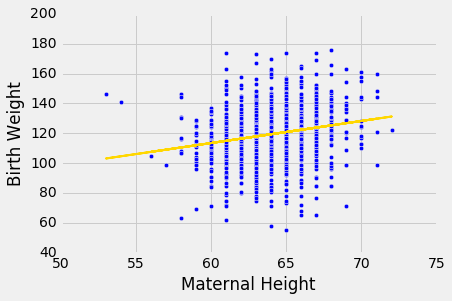``````correlation(baby, 'Maternal Height', 'Birth Weight')
0.20370417718968034
``````

``````bootstrap_slope(baby, 'Maternal Height', 'Birth Weight', 5000)
Slope of regression line: 1.47801935193
Approximate 95%-confidence interval for the true slope:
1.0403083964 1.91576886223
``````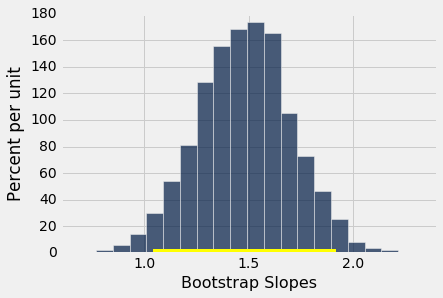### 真实斜率可能为 0 嘛？

``````draw_and_compare(0, 10, 25)
``````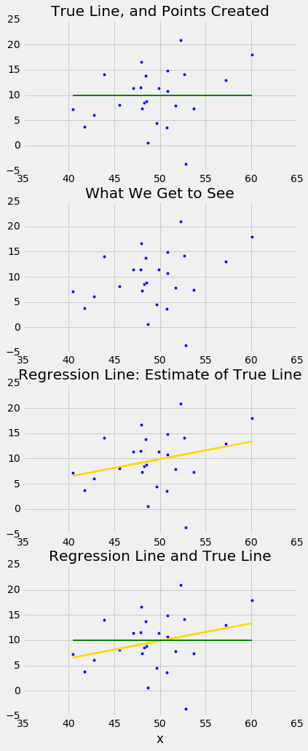``````slope(baby, 'Maternal Age', 'Birth Weight')
0.085007669415825132
``````

``````scatter_fit(baby, 'Maternal Age', 'Birth Weight')
``````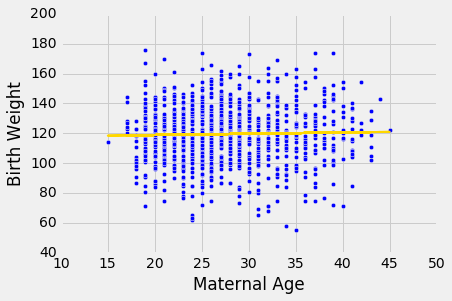``````bootstrap_slope(baby, 'Maternal Age', 'Birth Weight', 5000)
Slope of regression line: 0.0850076694158
Approximate 95%-confidence interval for the true slope:
-0.104335243815 0.272791852339
``````## 预测区间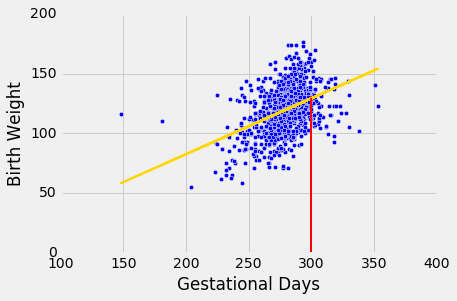``````def fitted_value(table, x, y, given_x):
a = slope(table, x, y)
b = intercept(table, x, y)
return a * given_x  + b
``````

``````fit_300 = fitted_value(baby, 'Gestational Days', 'Birth Weight', 300)
fit_300
129.2129241703143
``````

### 预测的可变性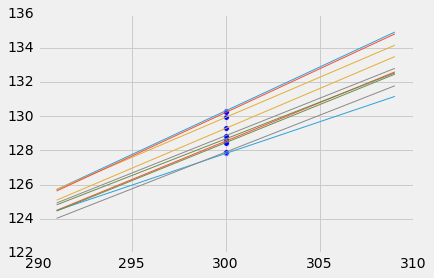``````lines
``````
slope intercept prediction at x=300
0.503931 -21.6998 129.479
0.53227 -29.5647 130.116
0.518771 -25.363 130.268
0.430556 -1.06812 128.099
0.470229 -11.7611 129.308
0.48713 -16.5314 129.608
0.51241 -23.2954 130.428
0.52473 -27.2053 130.214
0.409943 5.22652 128.21
0.468065 -11.6967 128.723

### 自举预测区间

• 表的名称
• 预测变量和响应变量的列标签
• 用于预测的`x`的值
• 所需的自举重复次数

``````# Bootstrap prediction of variable y at new_x
# Data contained in table; prediction by regression of y based on x
# repetitions = number of bootstrap replications of the original scatter plot

def bootstrap_prediction(table, x, y, new_x, repetitions):

# For each repetition:
# Bootstrap the scatter;
# get the regression prediction at new_x;
# augment the predictions list
predictions = make_array()
for i in np.arange(repetitions):
bootstrap_sample = table.sample()
bootstrap_prediction = fitted_value(bootstrap_sample, x, y, new_x)
predictions = np.append(predictions, bootstrap_prediction)

# Find the ends of the approximate 95% prediction interval
left = percentile(2.5, predictions)
right = percentile(97.5, predictions)

# Prediction based on original sample
original = fitted_value(table, x, y, new_x)

# Display results
Table().with_column('Prediction', predictions).hist(bins=20)
plots.xlabel('predictions at x='+str(new_x))
plots.plot(make_array(left, right), make_array(0, 0), color='yellow', lw=8);
print('Height of regression line at x='+str(new_x)+':', original)
print('Approximate 95%-confidence interval:')
print(left, right)
bootstrap_prediction(baby, 'Gestational Days', 'Birth Weight', 300, 5000)
Height of regression line at x=300: 129.21292417
Approximate 95%-confidence interval:
127.300774171 131.361729528
``````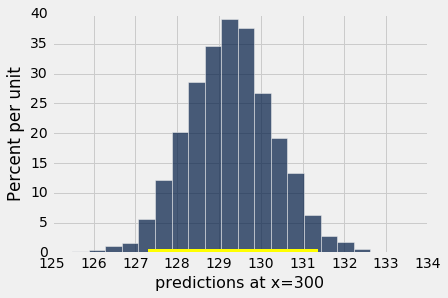### 改变预测变量的值的效果

``````bootstrap_prediction(baby, 'Gestational Days', 'Birth Weight', 285, 5000)
Height of regression line at x=285: 122.214571016
Approximate 95%-confidence interval:
121.177089926 123.291373304
``````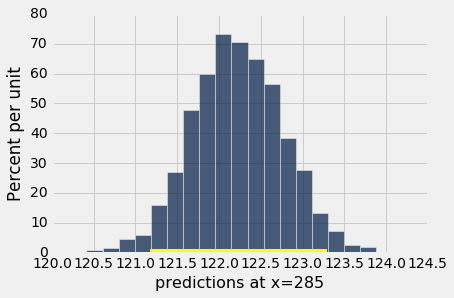``````np.mean(baby.column('Gestational Days'))
279.10136286201021
``````